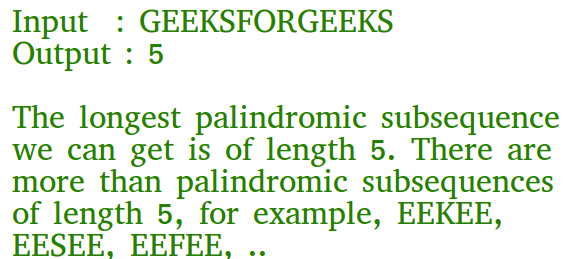# Java Program for Longest Palindromic Subsequence | DP-12

Given a sequence, find the length of the longest palindromic subsequence in it.As another example, if the given sequence is “BBABCBCAB”, then the output should be 7 as “BABCBAB” is the longest palindromic subseuqnce in it. “BBBBB” and “BBCBB” are also palindromic subsequences of the given sequence, but not the longest ones.
1) Optimal Substructure:
Let X[0..n-1] be the input sequence of length n and L(0, n-1) be the length of the longest palindromic subsequence of X[0..n-1].

If last and first characters of X are same, then L(0, n-1) = L(1, n-2) + 2.
Else L(0, n-1) = MAX (L(1, n-1), L(0, n-2)).
Following is a general recursive solution with all cases handled.

## Java

 `// Java program of above approach ` ` `  `class` `GFG { ` ` `  `    ``// A utility function to get max of two integers ` `    ``static` `int` `max(``int` `x, ``int` `y) ` `    ``{ ` `        ``return` `(x > y) ? x : y; ` `    ``} ` `    ``// Returns the length of the longest palindromic subsequence in seq ` ` `  `    ``static` `int` `lps(``char` `seq[], ``int` `i, ``int` `j) ` `    ``{ ` `        ``// Base Case 1: If there is only 1 character ` `        ``if` `(i == j) { ` `            ``return` `1``; ` `        ``} ` ` `  `        ``// Base Case 2: If there are only 2 characters and both are same ` `        ``if` `(seq[i] == seq[j] && i + ``1` `== j) { ` `            ``return` `2``; ` `        ``} ` ` `  `        ``// If the first and last characters match ` `        ``if` `(seq[i] == seq[j]) { ` `            ``return` `lps(seq, i + ``1``, j - ``1``) + ``2``; ` `        ``} ` ` `  `        ``// If the first and last characters do not match ` `        ``return` `max(lps(seq, i, j - ``1``), lps(seq, i + ``1``, j)); ` `    ``} ` ` `  `    ``/* Driver program to test above function */` `    ``public` `static` `void` `main(String[] args) ` `    ``{ ` `        ``String seq = ``"GEEKSFORGEEKS"``; ` `        ``int` `n = seq.length(); ` `        ``System.out.printf(``"The length of the LPS is %d"``, ` `                       ``lps(seq.toCharArray(), ``0``, n - ``1``)); ` `    ``} ` `} `

Output:

```The length of the LPS is 5
```

Dynamic Programming Solution

## Java

 `// A Dynamic Programming based Java ` `// Program for the Egg Dropping Puzzle ` `class` `LPS { ` ` `  `    ``// A utility function to get max of two integers ` `    ``static` `int` `max(``int` `x, ``int` `y) { ``return` `(x > y) ? x : y; } ` ` `  `    ``// Returns the length of the longest ` `    ``// palindromic subsequence in seq ` `    ``static` `int` `lps(String seq) ` `    ``{ ` `        ``int` `n = seq.length(); ` `        ``int` `i, j, cl; ` `        ``// Create a table to store results of subproblems ` `        ``int` `L[][] = ``new` `int``[n][n]; ` ` `  `        ``// Strings of length 1 are palindrome of lentgh 1 ` `        ``for` `(i = ``0``; i < n; i++) ` `            ``L[i][i] = ``1``; ` ` `  `        ``// Build the table. Note that the lower ` `        ``// diagonal values of table are ` `        ``// useless and not filled in the process. ` `        ``// The values are filled in a manner similar ` `        ``// to Matrix Chain Multiplication DP solution (See ` `        ``// https:// www.geeksforgeeks.org/matrix-chain-multiplication-dp-8/). ` `        ``// cl is length of substring ` `        ``for` `(cl = ``2``; cl <= n; cl++) { ` `            ``for` `(i = ``0``; i < n - cl + ``1``; i++) { ` `                ``j = i + cl - ``1``; ` `                ``if` `(seq.charAt(i) == seq.charAt(j) && cl == ``2``) ` `                    ``L[i][j] = ``2``; ` `                ``else` `if` `(seq.charAt(i) == seq.charAt(j)) ` `                    ``L[i][j] = L[i + ``1``][j - ``1``] + ``2``; ` `                ``else` `                    ``L[i][j] = max(L[i][j - ``1``], L[i + ``1``][j]); ` `            ``} ` `        ``} ` ` `  `        ``return` `L[``0``][n - ``1``]; ` `    ``} ` ` `  `    ``/* Driver program to test above functions */` `    ``public` `static` `void` `main(String args[]) ` `    ``{ ` `        ``String seq = ``"GEEKSFORGEEKS"``; ` `        ``int` `n = seq.length(); ` `        ``System.out.println(``"The lnegth of the lps is "` `+ lps(seq)); ` `    ``} ` `} ` `/* This code is contributed by Rajat Mishra */`

Output:

```The lnegth of the lps is 5
```

Please refer complete article on Longest Palindromic Subsequence | DP-12 for more details!

My Personal Notes arrow_drop_up

Article Tags :

Be the First to upvote.

Please write to us at contribute@geeksforgeeks.org to report any issue with the above content.